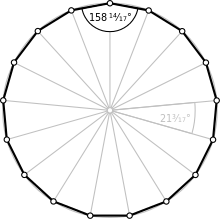Type Regular polygon
Edges and vertices 17
Schläfli symbol {17}
Coxeter diagram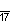Symmetry group Dihedral (D17), order 2×17
Internal angle (degrees) ≈158.82°
Dual polygon Self
Properties Convex, cyclic, equilateral, isogonal, isotoxal

In geometry, a heptadecagon or 17-gon is a seventeen-sided polygon.

A regular heptadecagon is represented by the Schläfli symbol {17}.

### Construction

As 17 is a Fermat prime, the regular heptadecagon is a constructible polygon (that is, one that can be constructed using a compass and unmarked straightedge): this was shown by Carl Friedrich Gauss in 1796 at the age of 19. This proof represented the first progress in regular polygon construction in over 2000 years. Gauss's proof relies firstly on the fact that constructibility is equivalent to expressibility of the trigonometric functions of the common angle in terms of arithmetic operations and square root extractions, and secondly on his proof that this can be done if the odd prime factors of n are distinct Fermat primes, which are of the form. Constructing a regular heptadecagon thus involves finding the cosine ofin terms of square roots, which involves an equation of degree 17—a Fermat prime. Gauss' book Disquisitiones Arithmeticae gives this as (in modern notation):Constructions for the regular triangle, pentagon, pentadecagon, and polygons with 2h times as many sides had been given by Euclid, but constructions based on the Fermat primes other than 3 and 5 were unknown to the ancients. (The only known Fermat primes are Fn for n = 0, 1, 2, 3, 4. They are 3, 5, 17, 257, and 65537.)

The explicit construction of a heptadecagon was given by Herbert William Richmond in 1893. The following method of construction uses Carlyle circles, as shown below. Based on the construction of the regular 17-gon, one can readily construct n-gons with n being the product of 17 with 3 or 5 (or both) and any power of 2: a regular 51-gon, 85-gon or 255-gon and any regular n-gon with 2h times as many sides.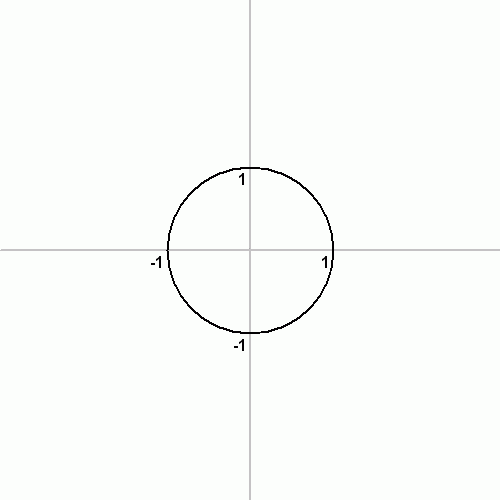Another construction of the regular heptadecagon using straightedge and compass is the following: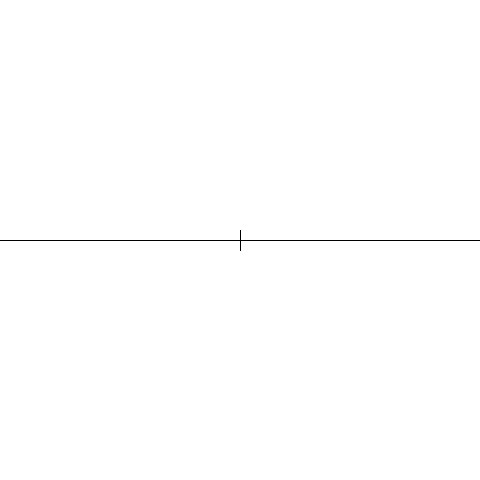The following simple design comes from Herbert William Richmond from the year 1893:

The point N3 is very close to the center point of Thales' theorem over AF.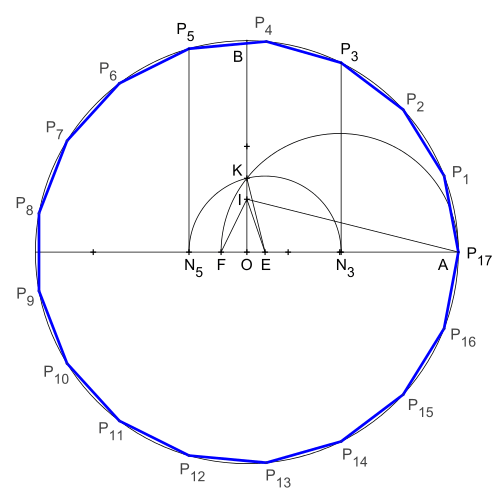Construction according to H. W. Richmond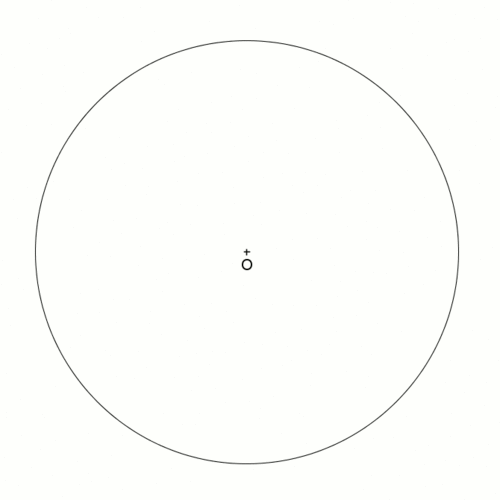Construction according to H. W. Richmond as animation

Another more recent construction is given by Callagy.

## Symmetry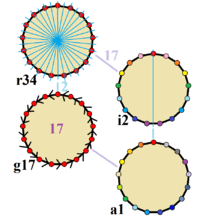Symmetries of a regular heptadecagon. Vertices are colored by their symmetry positions. Blue mirror lines are drawn through vertices and edges. Gyration orders are given in the center.

The regular heptadecagon has Dih17 symmetry, order 34. Since 17 is a prime number there is one subgroup with dihedral symmetry: Dih1, and 2 cyclic group symmetries: Z17, and Z1.

These 4 symmetries can be seen in 4 distinct symmetries on the heptadecagon. John Conway labels these by a letter and group order. Full symmetry of the regular form is r34 and no symmetry is labeled a1. The dihedral symmetries are divided depending on whether they pass through vertices (d for diagonal) or edges (p for perpendiculars), and i when reflection lines path through both edges and vertices. Cyclic symmetries in the middle column are labeled as g for their central gyration orders.

Each subgroup symmetry allows one or more degrees of freedom for irregular forms. Only the g17 subgroup has no degrees of freedom but can seen as directed edges.

## Related polygons

A heptadecagram is a 17-sided star polygon. There are seven regular forms given by Schläfli symbols: {17/2}, {17/3}, {17/4}, {17/5}, {17/6}, {17/7}, and {17/8}.

 Picture Interior angle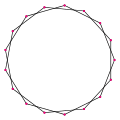{17/2}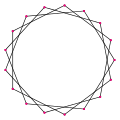{17/3}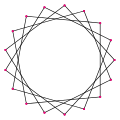{17/4}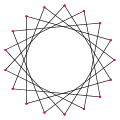{17/5}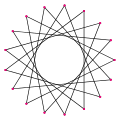{17/6}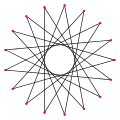{17/7}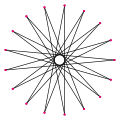{17/8} ≈137.647° ≈116.471° ≈95.2941° ≈74.1176° ≈52.9412° ≈31.7647° ≈10.5882°

### Petrie polygons

The regular heptadecagon is the Petrie polygon for one higher-dimensional regular convex polytope, projected in a skew orthogonal projection: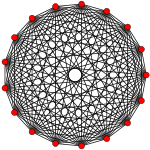16-simplex (16D)

## References

1. Arthur Jones, Sidney A. Morris, Kenneth R. Pearson, Abstract Algebra and Famous Impossibilities, Springer, 1991, ISBN 0387976612, p. 178.
2. Callagy, James J. "The central angle of the regular 17-gon", Mathematical Gazette 67, December 1983, 290–292.
3. Herbert W. Richmond, description "A Construction for a regular polygon of seventeen side" illustration (Fig. 6), The Quarterly Journal of Pure and Applied Mathematics 26: pp. 206–207, retrieved on 4th December 2015
4. John H. Conway, Heidi Burgiel, Chaim Goodman-Strauss, (2008) The Symmetries of Things, ISBN 978-1-56881-220-5 (Chapter 20, Generalized Schaefli symbols, Types of symmetry of a polygon pp. 275-278)